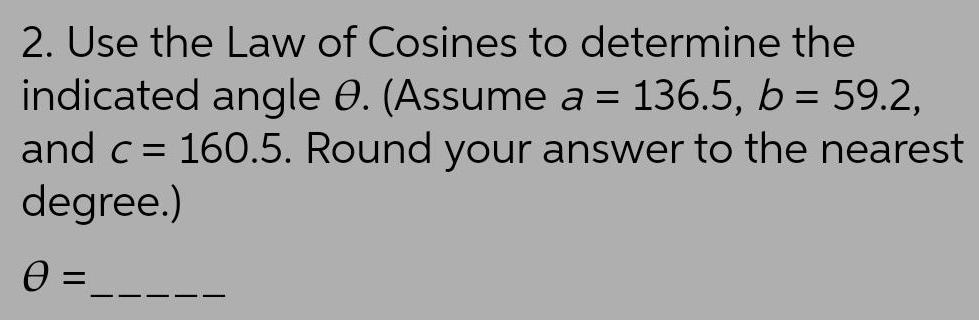Question:

# 2. Use the Law of Cosines to determine the indicated angle

Last updated: 7/6/20222. Use the Law of Cosines to determine the indicated angle O. (Assume a = 136.5, b = 59.2, and c = 160.5. Round your answer to the nearest degree.) 0 =